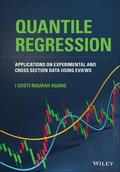John Wiley & Sons Quantile RegressionQUANTILE REGRESSION A thorough presentation of Quantile Regression designed to help readers obtain .. Product #: 978-1-119-71517-7 Regular price: \$101.87 \$101.87 Auf Lager

# Quantile Regression

## Applications on Experimental and Cross Section Data using EViews1. Auflage Juli 2021
496 Seiten, Hardcover
Wiley & Sons Ltd

ISBN: 978-1-119-71517-7
John Wiley & Sons

Jetzt kaufen

### Preis: 109,00 €

Preis inkl. MwSt, zzgl. Versand

QUANTILE REGRESSION

A thorough presentation of Quantile Regression designed to help readers obtain richer information from data analyses

The conditional least-square or mean-regression (MR) analysis is the quantitative research method used to model and analyze the relationships between a dependent variable and one or more independent variables, where each equation estimation of a regression can give only a single regression function or fitted values variable. As an advanced mean regression analysis, each estimation equation of the mean-regression can be used directly to estimate the conditional quantile regression (QR), which can quickly present the statistical results of a set nine QR(Ä)s for Ä(tau)s from 0.1 up to 0.9 to predict detail distribution of the response or criterion variable. QR is an important analytical tool in many disciplines such as statistics, econometrics, ecology, healthcare, and engineering.

Quantile Regression: Applications on Experimental and Cross Section Data Using EViews provides examples of statistical results of various QR analyses based on experimental and cross section data of a variety of regression models. The author covers the applications of one-way, two-way, and n-way ANOVA quantile regressions, QRs with multi numerical predictors, heterogeneous QRs, and latent variables QRs, amongst others. Throughout the text, readers learn how to develop the best possible quantile regressions and how to conduct more advanced analysis using methods such as the quantile process, the Wald test, the redundant variables test, residual analysis, the stability test, and the omitted variables test. This rigorous volume:
* Describes how QR can provide a more detailed picture of the relationships between independent variables and the quantiles of the criterion variable, by using the least-square regression
* Presents the applications of the test for any quantile of any numerical response or ­criterion variable
* Explores relationship of QR with heterogeneity: how an independent variable affects a dependent variable
* Offers expert guidance on forecasting and how to draw the best conclusions from the results obtained
* Provides a step-by-step estimation method and guide to enable readers to conduct QR analysis using their own data sets
* Includes a detailed comparison of conditional QR and conditional mean regression

Quantile Regression: Applications on Experimental and Cross Section Data Using EViews is a highly useful resource for students and lecturers in statistics, data analysis, econometrics, engineering, ecology, and healthcare, particularly those specializing in regression and quantitative data analysis.

Ch. 1: Test for Equality of Medians by Series/Group OF Variables

Ch. 2: One and Two-Way ANOVA Quantile Regressions

Ch. 3: N-Way ANOVA Quantile Regressions

Ch. 4: Quantile Regressions Based On (Xi,Yi)

Ch. 5: Quantile Regressions with Two Numerical Predictors

Ch. 6: Quantile Regressions with Multi Numerical Predictors

Ch. 7: Quantile Regressions with the Ranks of Numerical Predictors

Ch. 8: Heterogeneous Quantile Regressions based on Experimental Data

Ch. 9: Quantile Regressions Based On CPS88.wf1

Ch.10 : QUANTILE REGRESSIONS OF A LATENT VARIABLE

Appendix A

Appendix B

Appendix C

Bibliography
I Gusti Ngurah Agung, PhD, The Ary Suta Center, Jakarta, Indonesia. Professor Agung taught at the State University of Makassar from 1960-1987, and at the University of Indonesia from 1987-2018. His research has focused on finding expected or unpredictable statistical results based on various models of time series, cross-section, experimental data, and panel data models. In addition, he is also interested in cross-section data over times and a special or uncommon panel data in indexes.

Agung, State University of Makassar; University of Indonesia